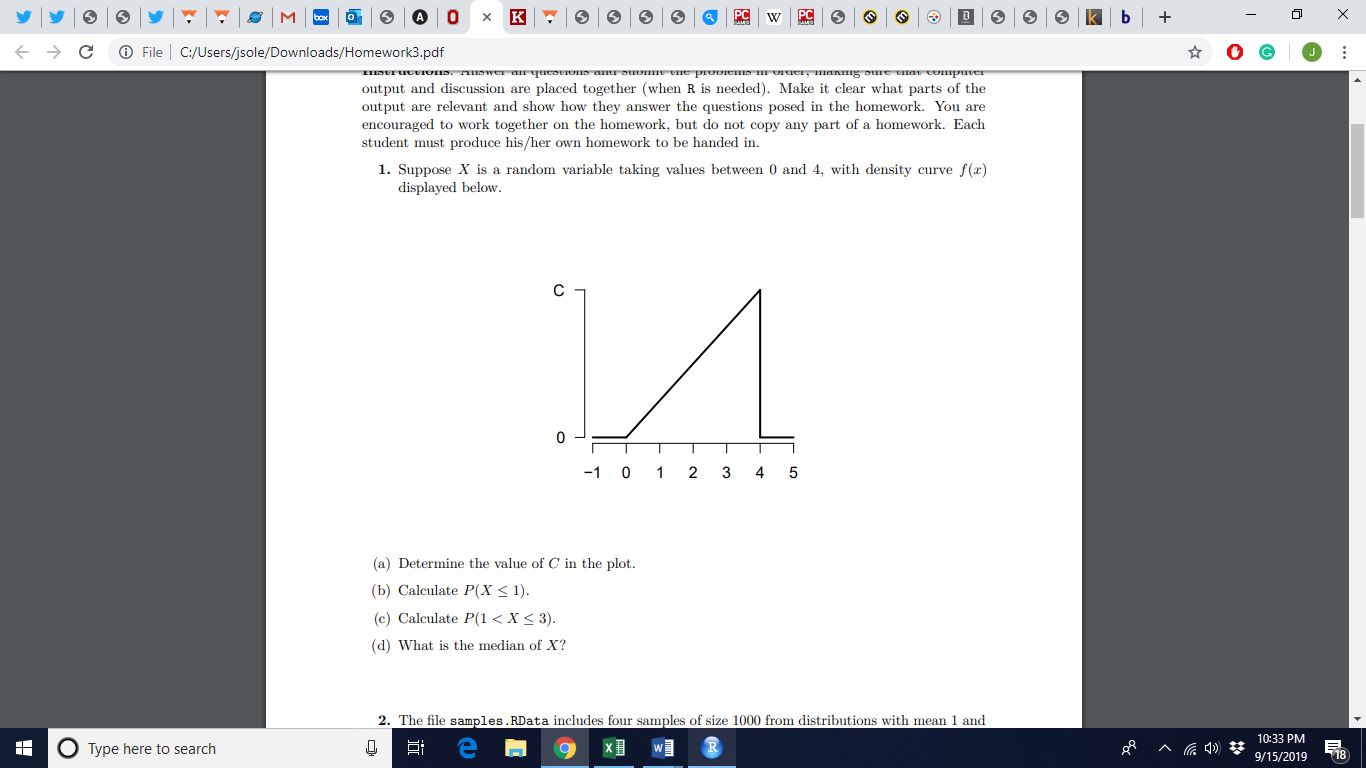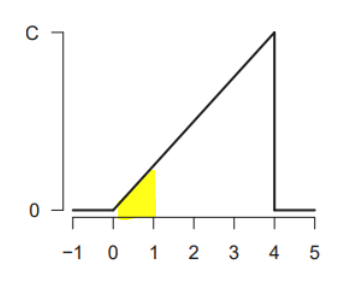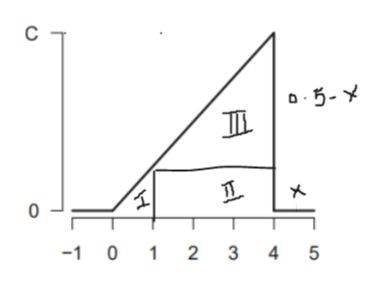0+File C:/Users/jsole/Downloads/Homework3.pdfCzaanduma am ame Suvu1 iranin I emanaid ama aane n enAmcanh m aMem eHAranneuoutput and discussion are placed together (when R is needed). Make it clear what parts of theoutput are relevant and show how they answer the questions posed in the homework. You areencouraged to work together on the homework, but do not copy any part of a homework. Eachstudent must produce his/her own homework to be handed in1. Suppose X is a random variable taking values between 0 and 4, with density curve f (x)displayed belowC234-1 0 15(a) Determine the value of C in the plot.(b) Calculate P(X < 1).(c) Calculate P(1 < X < 3)(d) What is the median of X?2. The file samples.RData includes four samples of size 1000 from distributions with mean 1 and10:33 PM9/15/2019O Type here to search18

Question

Hello,

Please find attached the question I would like help on. Specifically part b.

I have already figured out the answer to part a is 1/2 and I think if I can figure out part b, I can do part c.help_outlineImage Transcriptionclose0 + File C:/Users/jsole/Downloads/Homework3.pdf C zaanduma am ame Suvu1 iranin I emanaid ama aane n enAmcanh m aMem eHAranneu output and discussion are placed together (when R is needed). Make it clear what parts of the output are relevant and show how they answer the questions posed in the homework. You are encouraged to work together on the homework, but do not copy any part of a homework. Each student must produce his/her own homework to be handed in 1. Suppose X is a random variable taking values between 0 and 4, with density curve f (x) displayed below C 2 3 4 -1 0 1 5 (a) Determine the value of C in the plot. (b) Calculate P(X < 1). (c) Calculate P(1 < X < 3) (d) What is the median of X? 2. The file samples.RData includes four samples of size 1000 from distributions with mean 1 and 10:33 PM 9/15/2019 O Type here to search 18 fullscreen
Step 1

a)

Total area under the curve is a triangle with area = 1/2 * base * height = 1/2 * 4 * C = 2C

Since you've figured out part a), you must already know that the total area under the density curve = 1

Therefore, 2C = 1 which gives us C=1/2

Step 2

b)

P(x<=1) means percent of area under the density curve which is less than or equal to 1 as shown by yellow triangle.

To calculate the area of the yellow traingle, we need its height which can be calculated by splitting the total areas into 3 parts.help_outlineImage TranscriptioncloseИ C -1 0 1 2 3 4 5 fullscreen
Step 3

Area of region I = 1/2 * (1-0)* x  = 0.5x

Area of region 2 = (4-1)*x = 3x

Area of region 3 = 1/2 *(4-1)*(0.5-x) = 0.75-1.5x

Sum of all three areas must be equal to 1

Therefore,  0.5 x + 3 x + 0.75 -1.5x =1  or

&nbs...help_outlineImage TranscriptioncloseC D5-Y -1 0 1 2 3 4 5 fullscreen

Want to see the full answer?

See Solution

Want to see this answer and more?

Our solutions are written by experts, many with advanced degrees, and available 24/7

See Solution
Tagged in

Advanced Topics in Statistics